# 第三次作业

1.实验代码：

#include<stdio.h>
int main(){
int a=1,sum;
sum = 0;
for (a = 1; a <= 100; a++)
sum = a + sum;
printf("sum = %d\n", sum);
system("pause");
return 0;
}

2.解题思路：

（1）

（2）流程图：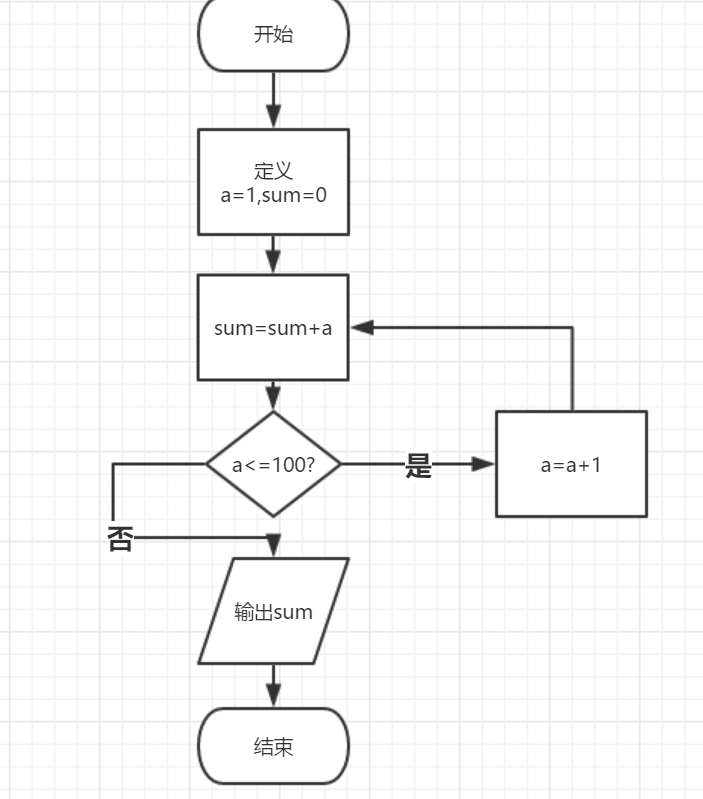3.本题调试过程碰到问题及解决办法：

运行结果截图：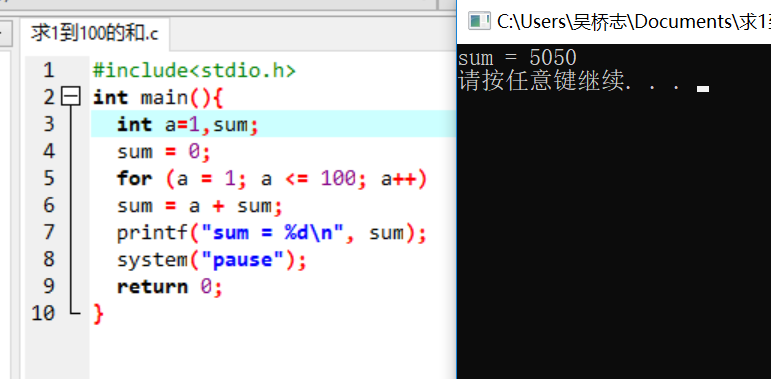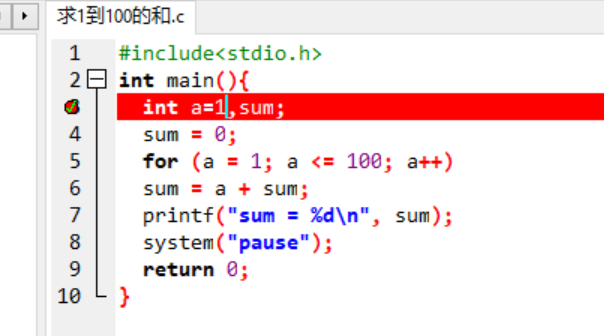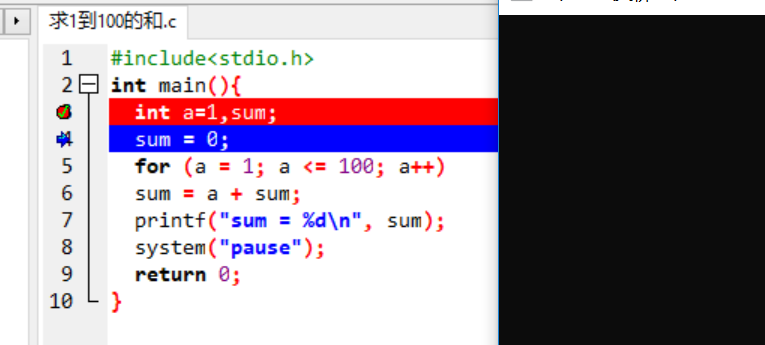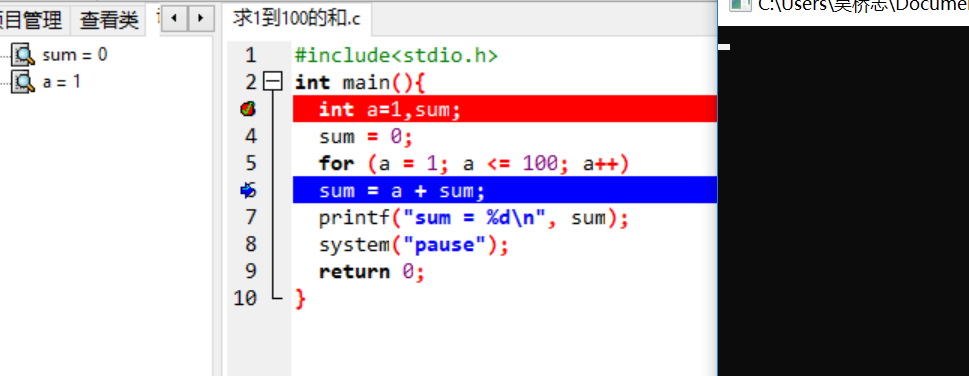1.实验代码

#include<stdio.h>
int main(){
int a,t=0;
while(a=1)
{
scanf("%d",&a);
t++;
if(a==250)
{
printf("%d\n",t);
break;
}
}
return 0;
}

2.解题思路：

（1）

（2）流程图：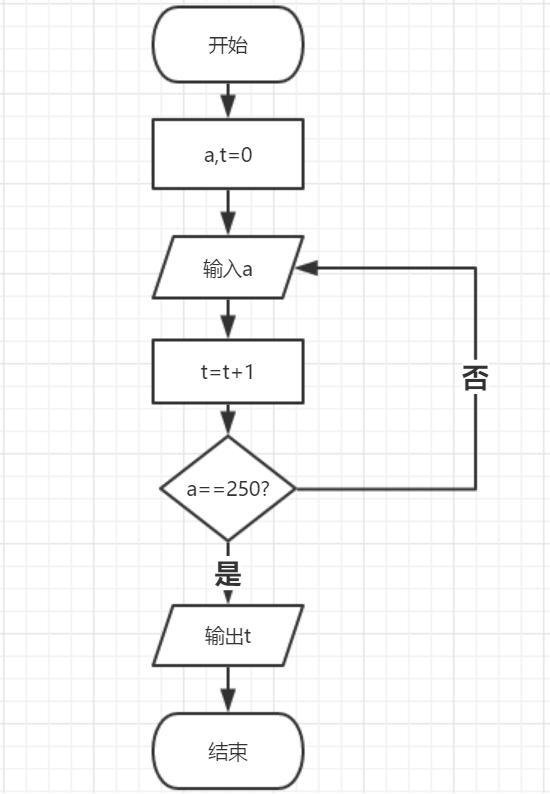3.本题调试过程碰到问题及解决办法：

运行结果截图：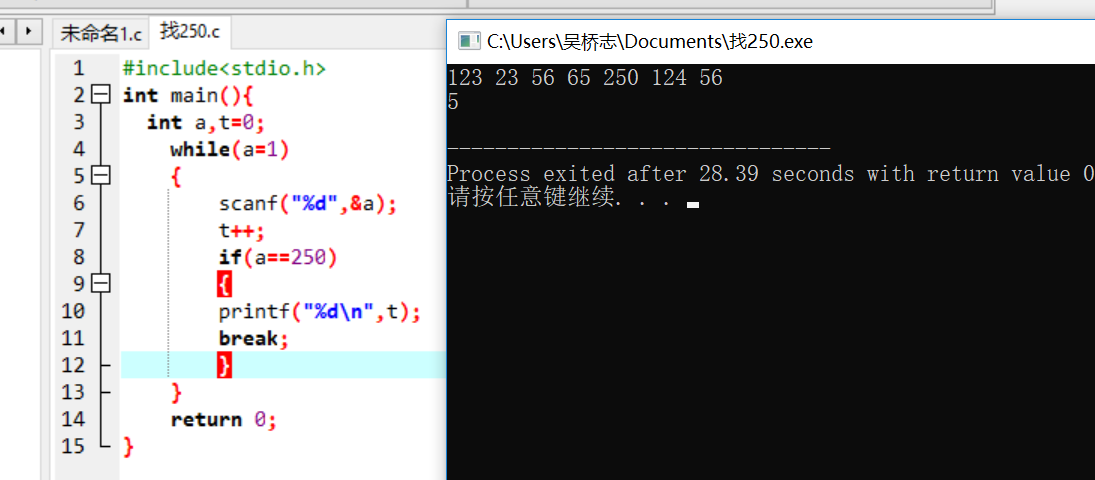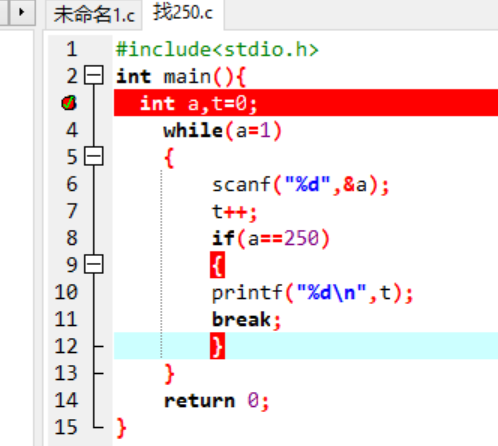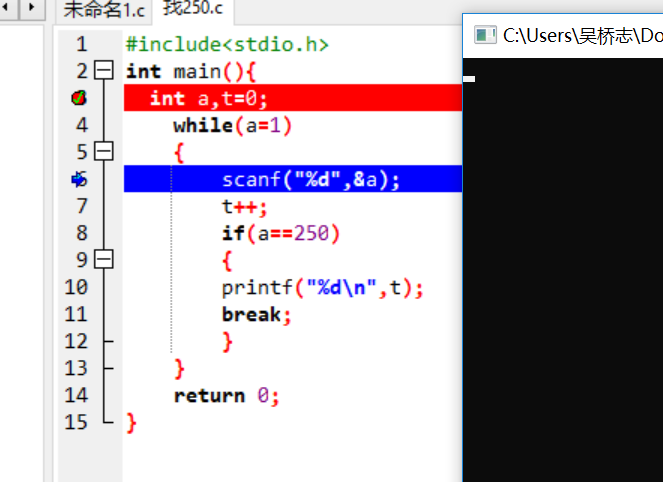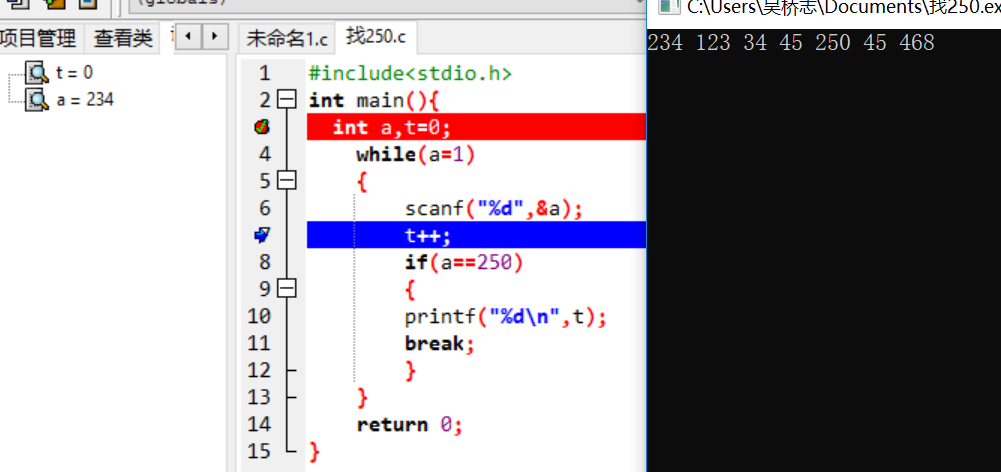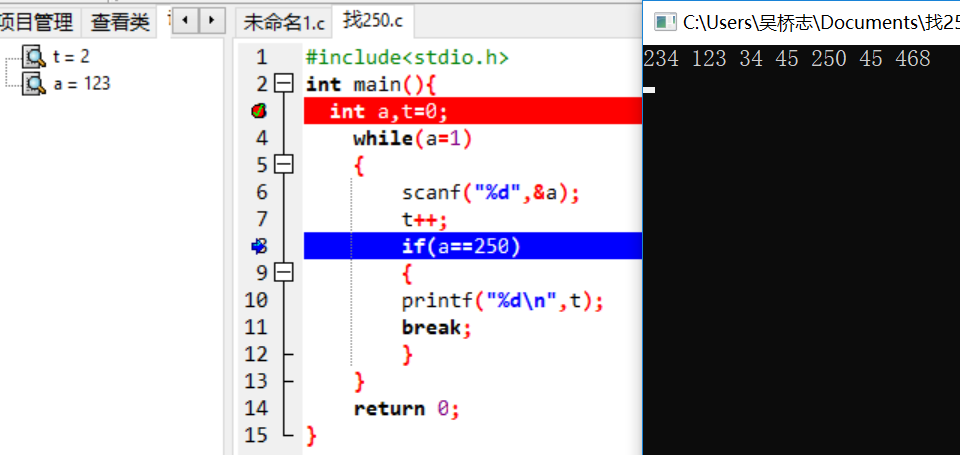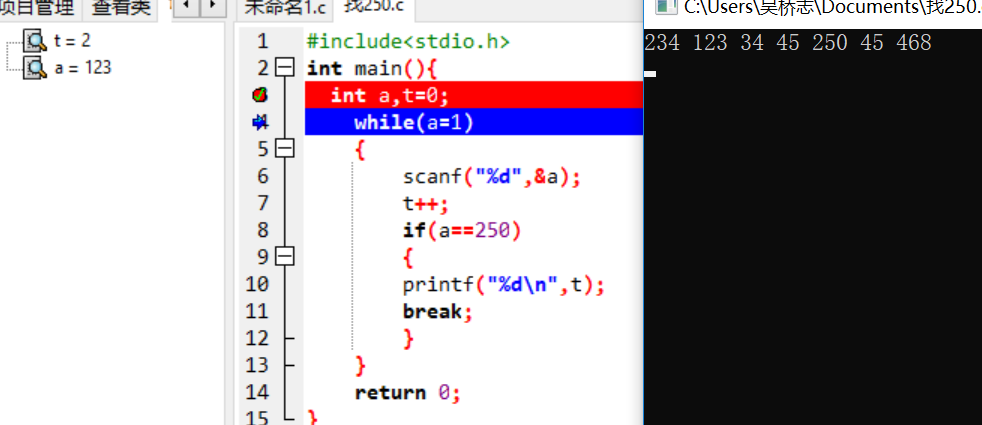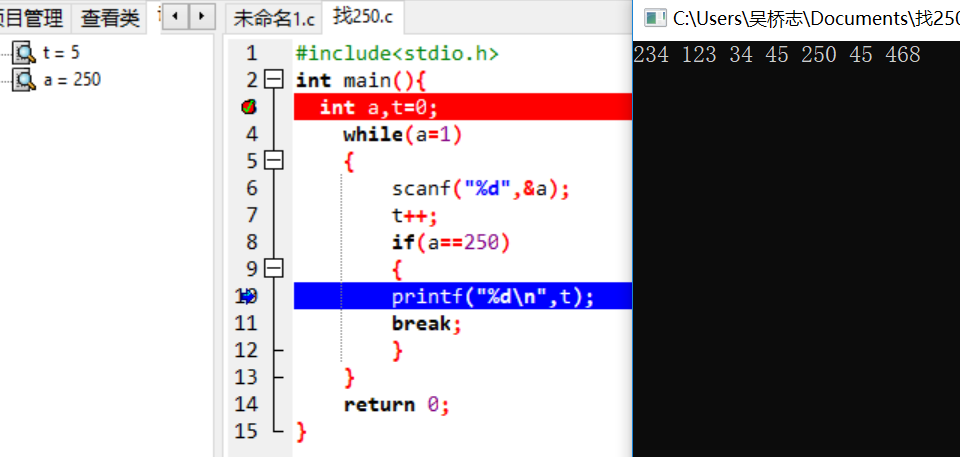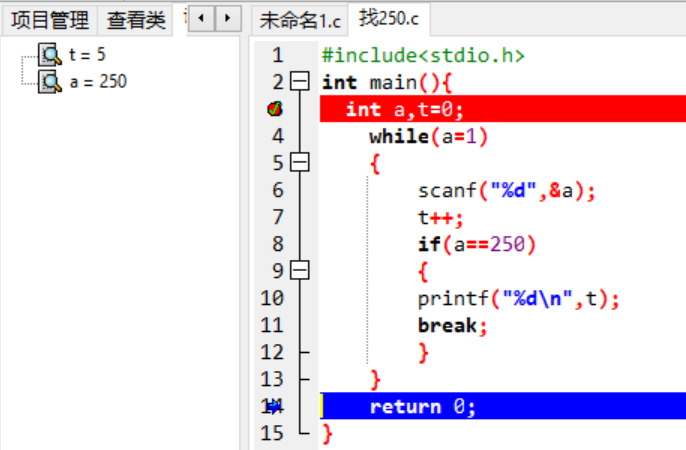在答题过程中，我把t++放到了if语句里面了，伴随着输入的数字不同，t的值也一直在变，经过调试发现，当输入的值不是250，t的值就不再变化，于是我把t++放在if语句的前面，最后解决了这个问题。

个人总结：

第十次作业：题目7-4 最大公约数和最小公倍数

1.实验代码：

#include<stdio.h>int main(){ int m,i,n,w=0,j; scanf("%d %d",&m,&n); if(m>n) {       w=m;  m=n;  n=w; } for(i=m;i>=1;i--){   if(m%i==0&&n%i==0)   {   break; } } for(j=n;j<=m*n;j++){      if(j%m==0&&j%n==0){   break; } }    printf("%d %d\n",i,j); return 0;}

2.解题思路：

（1）

（2）流程图：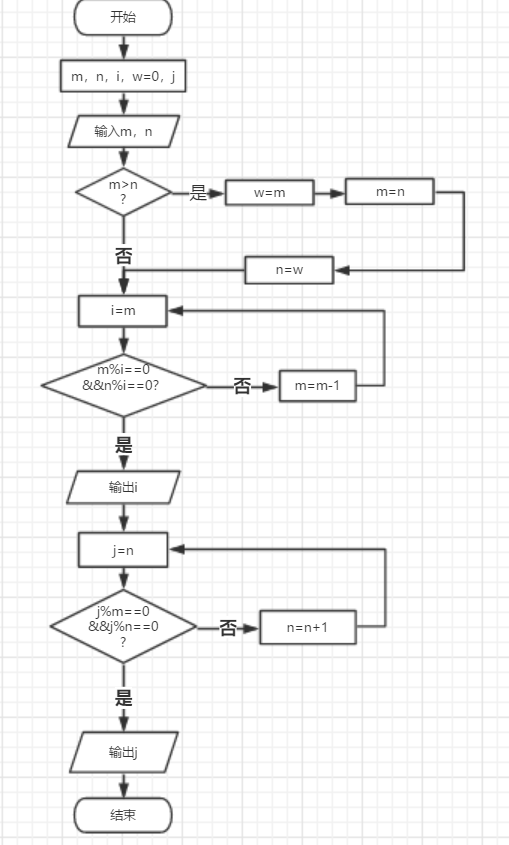3.本题调试过程碰到问题及解决办法：

运行结果截图：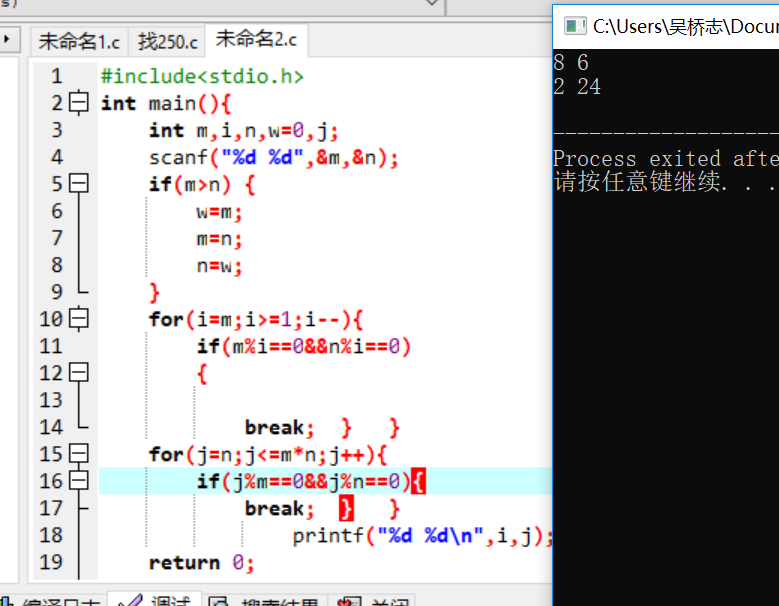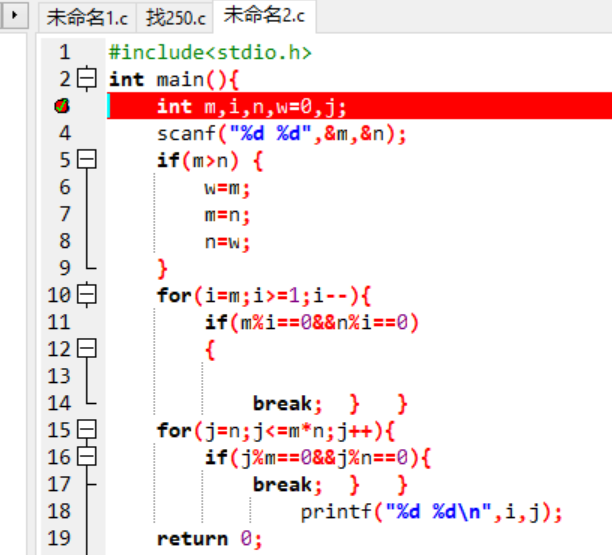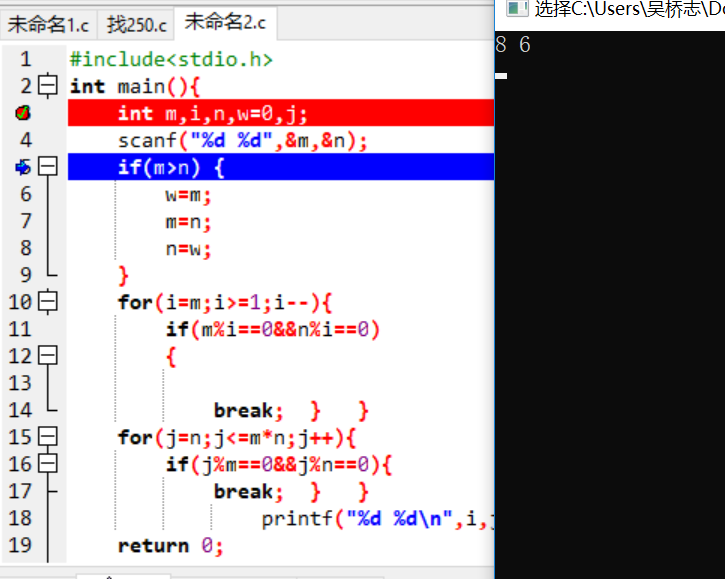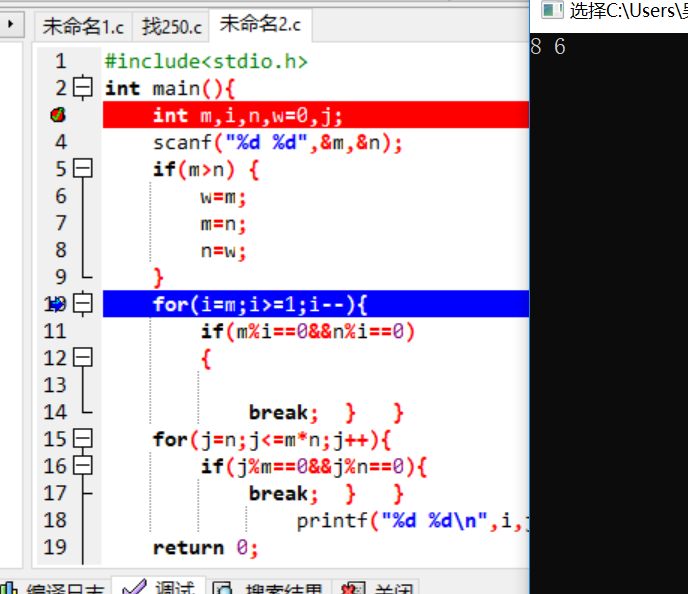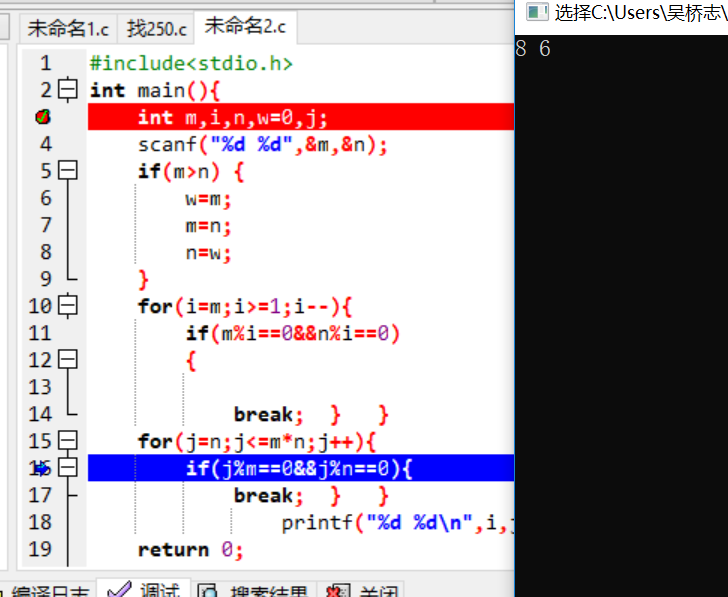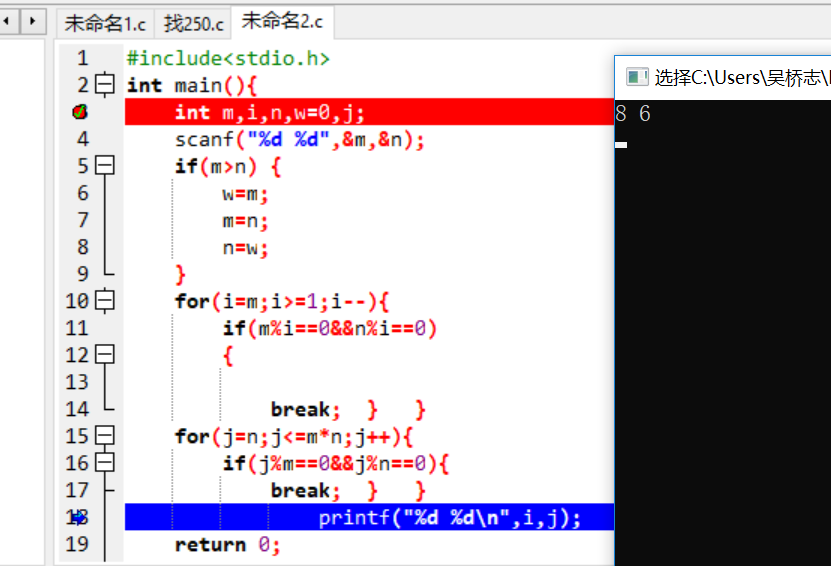个人总结：

学习进度：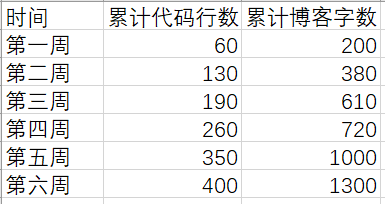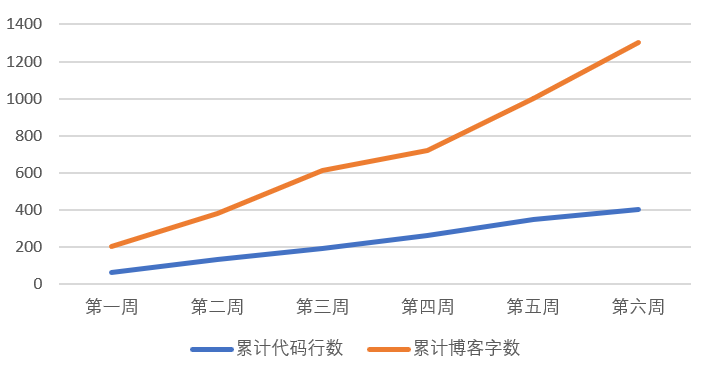互评：

posted @ 2018-11-28 23:12  吴桥志  阅读(154)  评论(6编辑  收藏  举报